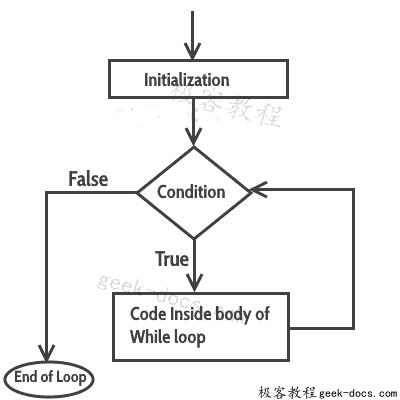# C++ while循环

#### while循环的语法

while(condition)
{
statement(s);
}


## 循环如何工作？

while循环中，首先计算条件，如果它返回true，则执行while循环中的语句，这会重复发生，直到条件返回false。当条件返回false时，控制流退出循环并跳转到程序中的while循环后的下一个语句。

### while循环流程图## C++中的while循环示例

#include <iostream>
using namespace std;
int main(){
int i=1;
/* The loop would continue to print
* the value of i until the given condition
* i<=6 returns false.
*/
while(i<=6){
cout<<"Value of variable i is: "<<i<<endl; i++;
}
}


Value of variable i is: 1
Value of variable i is: 2
Value of variable i is: 3
Value of variable i is: 4
Value of variable i is: 5
Value of variable i is: 6


## 无限循环

#include <iostream>
using namespace std;
int main(){
int i=1; while(i<=6) {
cout<<"Value of variable i is: "<<i<<endl; i--;
}
}


## 示例：使用while循环显示数组元素

#include <iostream>
using namespace std;
int main(){
int arr[]={21,87,15,99, -12};
/* The array index starts with 0, the
* first element of array has 0 index
* and represented as arr
*/
int i=0;
while(i<5){
cout<<arr[i]<<endl;
i++;
}
}


21
87
15
99
-12



• 回顶